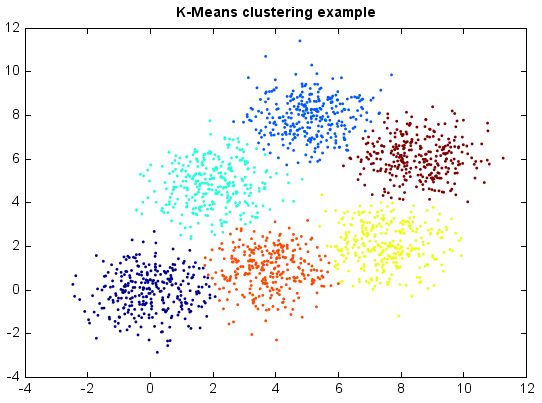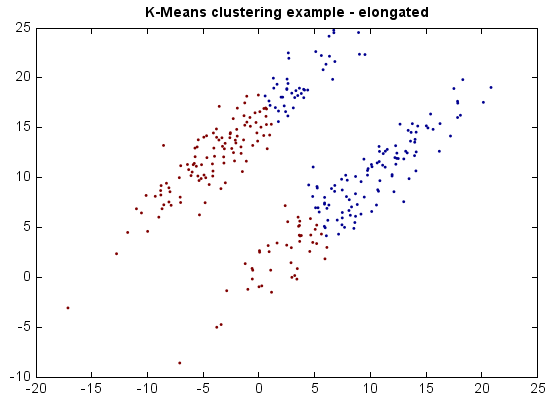# K-Means¶

K-Means clustering partitions n observations into k clusters in which each observation belongs to the cluster with the nearest mean. Although finding an exact solution to the K-Means problem for arbitrary input is NP-hard, the standard approach to finding an approximate solution (often called Lloyd’s algorithm or the K-Means algorithm) is used widely and frequently finds reasonable solutions quickly.

K-Means is a hard clustering method, i.e. each sample is assigned to a specific cluster. In contrast, soft clustering, e.g. the Expectation-Maximization algorithm for Gaussian mixtures, assign samples to different clusters with different probabilities.

K-Means works very well on Gaussian mixtures.

```from miml import datasets
from miml.cluster import KMeans

fn = os.path.join(datasets.get_data_home(), 'clustering', 'gaussian',
'six.txt')
format='%2f')
x = df.values

model = KMeans(6, runs=20)
y = model.fit_predict(x)

scatter(x[:,0], x[:,1], c=y, edgecolor=None, s=3)
title('K-Means clustering example')
```If the clusters are elongated, however, the results may be far from optimal.

```from miml import datasets
from miml.cluster import KMeans

fn = os.path.join(datasets.get_data_home(), 'clustering', 'gaussian',
'elongate.txt')